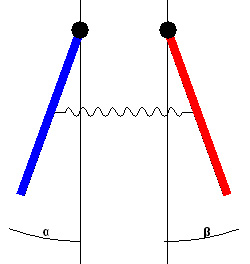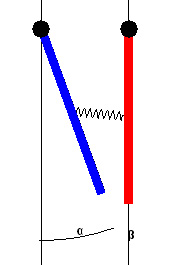# Coupled Pendulums

Oscillations of Coupled pendula is an interesting phenomenon in Physics.

A one dimensional oscillation can be thought of as an object moving back and forth about an equilibrium position. In this experiment we consider the oscillating system of two coupled pendulums (they are coupled by springs).

If we connect two identical pendulums with a spring, one is held by hand, and another is deviated aside so the spring is stressed and then both the pendulums are released. How they will move? Intuitively it seems that the still pendulum will set into the motion under action of a spring and after a while both pendulums will oscillate chaotically.  Actually Something very interesting happens.

### The pendulums will periodically exchange the energy and stop by turns.

In this experiment we can study this periodic “swapping” of energy in two identical pendulums coupled with a spring.  If we swing one pendulum, then the oscillatory energy will begin to be transferred to other pendulum. After the end of this process the energy will be transferred in the opposite direction. Thus, there is a periodic exchange of energy between the pendulums. As a result the amplitude of oscillation of every pendulum is periodically changed. When one pendulum oscillate with the maximal amplitude the other one is still.

Here is the experimental set-up….
Pendulums are fixed to DC motors (we are using these as pick-up device as rotational motion sensors are very costly). The emf generated is amplified amplifier in ExpEYES and is fed to channels A1 and A2. Using voltage capture functions plots are obtained.

The waveforms generated using DC motors after amplification…

Figure 1 shows waveform for the first pendulum and Figure 2  shows waveform for the second pendulum. In figure 3 both waveforms are plotted together.

Using subplot function of matplotlib the following figure is obtained. Here one can easily compare the waveforms of two pendulums.The waveforms show beats as theoretically expected….

This experiment can be performed by oscillating pendulums in different ways…

• Both oscillating in phase
• Both oscillating out of phase
• One at rest and other oscillating

When both the pendulums oscillate in phaseWhen  pendulums oscillate out of phaseWhen one pendulum is at rest while the other is given oscillations## What is really happening?

{When one pendulum is at rest while the other is set in to oscillations}
Every pendulum has a natural or resonant frequency, which is the number of times the pendulum swings back and forth per second. The resonant frequency depends on the pendulum’s length. Longer pendulums have lower frequencies.Every time the first pendulum swings, it pulls on the connecting spring and gives the second pendulum a small tug. Since the two pendulums have the same length, they have the same natural frequency.  Therefore the second pendulum begins to swing. The second pendulum swings slightly out of phase with the first one. That is, when the first pendulum is at the height of its swing, the second pendulum is still somewhere in the middle of its swing. As soon as the second pendulum starts to swing, it starts pulling back on the first pendulum. These pulls are timed so that the first pendulum slows down. To picture this, it may help you to think of a playground swing. When you push on the swing at just the right moments, it goes higher and higher. When you push the swing at just the wrong moments, it slows down and stops.The second pendulum pulls on the first pendulum at just the “wrong” moments. Eventually, the first pendulum is brought to rest; it has transferred all of its energy to the second pendulum. But now the original situation is exactly reversed, and the first pendulum is in a position to begin stealing energy back from the second. And so it goes, the energy repeatedly switching back and forth until friction and air resistance finally steal all of it away from both pendulums.If the two pendulums are not the same length, then the tugs from the first pendulum’s swings will not occur at the natural frequency of the second one. The two pendulums swing but with an uneven, jerky motion.